Vectors and conics

This free course is available to start right now. Review the full course description and key learning outcomes and create an account and enrol if you want a free statement of participation.

Free course

3.4 Further exercises

Example 47

• (a) Find the angle between each of the pairs of vectors:

(3, 1) and (1, −2); i + 2j and −3i + j − 2k.

• (b) Determine the projection of (3, 1) onto (1, −2), and the projection of i + 2j onto −3i + j − 2k.

Denote by p the first vector of each pair, by q the second vector and by θ the angle between the vectors.

• (a) When p = (3, 1) and q = (1, −2), we have

•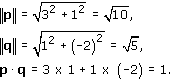• It follows that

•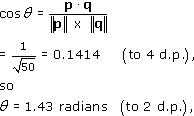• When p = i + 2j and q = −3i + j − 2k, we have

•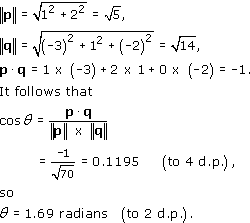• (b) When p = (3, 1) and q = (1, −2), the projection of p onto q is

•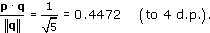• When p = i + 2j and q = −3i + j − 2k, the projection of p onto q is

•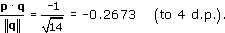Example 48

Determine the two vectors of length 2 which make an angle of/4 with the vector p = (2, −2). Verify that the two vectors that you have found are perpendicular to each other.

Let such a vector be r = (x, y). Since r has length 2 and makes an angle/4 with p = (2, −2),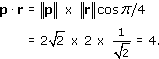In component form we have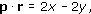so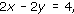that is,

x = y + 2.

Also, r has length 2, so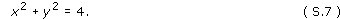Substituting the expression for x into equation (S.7), we obtain

(y + 2)2 + y2 = 4,

thus

y2 + 4y + 4 + y2 = 4,

that is,

2y2 + 4y = 0.

This has solutions

y = 0 and y = −2;

the corresponding values of x are x = 2 and x = 0.

It follows that the two vectors of length 2 making an angle of/4 with (2, −2) are (2, 0) and (0, −2).

The dot product of these two vectors is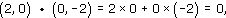so the two vectors are perpendicular.

Example 49

Determine the equation of the plane that contains the point (−1, 3, 2) with (1, 2, −1) as a normal.

We use the corollary to Theorem 1. The equation of the plane is given by x · n = p · n, where x = (x, y, z), p = (−1, 3, 2) and n = (1, 2, −1).

Thus the equation of the plane is

x + 2y − z = (−1) × 1 + 3 = 2 + 2 = (−1),

that is,

x + 2y − z = 3.

Example 50

Determine the equation of the plane through (1, 0, 2), (0, 3, 4) and (0, −1, 0).

Let the equation of the plane be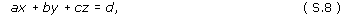for some real numbers a, b, c and d, where a, b and c are not all zero.

Substituting the coordinates of the three points in turn into equation (S.8), we obtain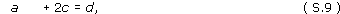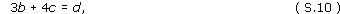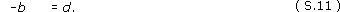Substituting −b for d in equations (S.9) and (S.10), we obtain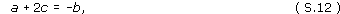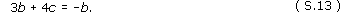Equation (S.13) gives c = −b; if we then substitute −b for c in equation (S.12), we obtain

a − 2b = −b,

that is,

a = b.

It follows that the equation of the plane is

bx + by − bz = −b,

that is,

x + y − z = −1.

M208_1